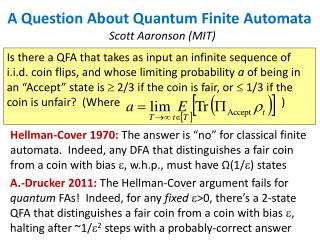DownloadDownload PresentationA Question About Quantum Finite Automata

# A Question About Quantum Finite Automata

Download Presentation## A Question About Quantum Finite Automata

- - - - - - - - - - - - - - - - - - - - - - - - - - - E N D - - - - - - - - - - - - - - - - - - - - - - - - - - -
##### Presentation Transcript

1. Is there a QFA that takes as input an infinite sequence of i.i.d. coin flips, and whose limiting probability a of being in an “Accept” state is  2/3 if the coin is fair, or  1/3 if the coin is unfair? (Where ) A Question About Quantum Finite Automata Hellman-Cover 1970: The answer is “no” for classical finite automata. Indeed, any DFA that distinguishes a fair coin from a coin with bias , w.h.p., must have Ω(1/) states Scott Aaronson (MIT) A.-Drucker 2011: The Hellman-Cover argument fails for quantum FAs! Indeed, for any fixed>0, there’s a 2-state QFA that distinguishes a fair coin from a coin with bias , halting after ~1/2 steps with a probably-correct answer

2. Idea of the QFA for fixed : Just rotate a qubit an () amount clockwise with each heads, or counterclockwise with each tails With (2) probability, measure in {|0,|1} basis On the other hand: let p = coin bias and S = dimension of the QFA. Then Drucker and I showed that the limiting acceptance probability a(p) is a quotient g(p)/h(p) of two degree-S2 polynomials, except possibly when h(p)=0 (and that’s the trouble!) a(p) p

3. Now, if the QFA halts on entering an Accept state, then we can show that a(p) is a rational function on the entire open intervalp(0,1) (Though possibly not at the endpoints—do you see why?) So, at least in the halting case, a single QFA indeed can’t distinguish p=1/2 from all p1/2 My question is whether this can be extended to QFAs that never halt, but only “accept” or “reject” in the limit